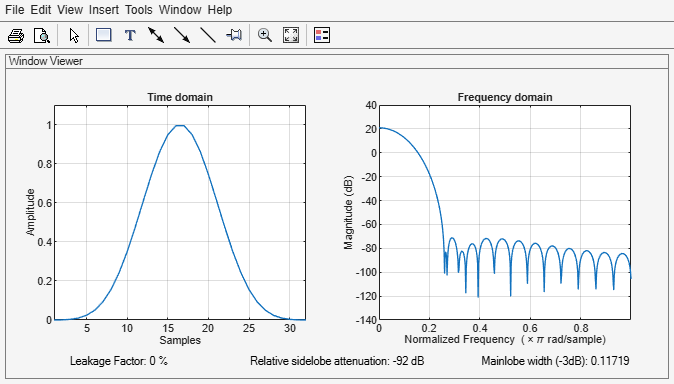# blackmanharris

Minimum four-term Blackman-Harris window

## Syntax

``w = blackmanharris(N)``
``w = blackmanharris(N,sflag)``

## Description

example

````w = blackmanharris(N)` returns an `N`-point symmetric four-term Blackman-Harris window.```
````w = blackmanharris(N,sflag)` returns a Blackman-Harris window using the window sampling method specified by `sflag`.```

## Examples

collapse all

Create a 32-point symmetric Blackman-Harris window. Display the result using `wvtool`.

```N = 32; wvtool(blackmanharris(N))```## Input Arguments

collapse all

Window length, specified as a positive integer.

Data Types: `single` | `double`

Window sampling method, specified as:

• `'symmetric'` — Use this option when using windows for filter design.

• `'periodic'` — This option is useful for spectral analysis because it enables a windowed signal to have the perfect periodic extension implicit in the discrete Fourier transform. When `'periodic'` is specified, the function computes a window of length `L` + 1 and returns the first `L` points.

## Output Arguments

collapse all

Blackman-Harris window, returned as a column vector.

## Algorithms

The equation for the symmetric four-term Blackman-Harris window of length N is

`$w\left(n\right)={a}_{0}-{a}_{1}\mathrm{cos}\left(\frac{2\pi n}{N-1}\right)+{a}_{2}\mathrm{cos}\left(\frac{4\pi n}{N-1}\right)-{a}_{3}\mathrm{cos}\left(\frac{6\pi n}{N-1}\right),\text{ }0\le n\le N-1$`

The equation for the periodic four-term Blackman-Harris window of length N is

`$w\left(n\right)={a}_{0}-{a}_{1}\mathrm{cos}\frac{2\pi n}{N}+{a}_{2}\mathrm{cos}\frac{4\pi n}{N}-{a}_{3}\mathrm{cos}\frac{6\pi n}{N},\text{ }0\le n\le N-1$`

The periodic window is N-periodic.

CoefficientValue
a00.35875
a10.48829
a20.14128
a30.01168

 harris, fredric j. “On the Use of Windows for Harmonic Analysis with the Discrete Fourier Transform.” Proceedings of the IEEE®. Vol. 66, January 1978, pp. 51–83.